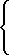Applets:  - DEW1  - DEWn Instructions:  - About  - DEW1  - DEWn  - Equation parser

# DEWn

DEWn draws the solution of differential equation of second and higher order with initial values t0 and y0. Also the phase plane of two chosen components is drawn. If the equation to be drawn is of second order then the direction field is drawn. Highest possible order of equation is 12.

## Drawing (Initial values)

Solution is drawn with the initial values t and y0, y1, y2... Values can be written to t= and y0=, y1=, y2= ... textfields and clicking the Draw -button. Or values can be pointed with a mouse from time dependency -area. If the equation is of second order then the values can also be pointed from the phase plane. The value of t can also be changed by writing a new value to textfield and pressing the Ok -button.

Components to be drawn can be chosen with checkboxes y0, y1, y2... By default each one is drawn.

## Equation

Differential equation may be selected from pre-defined examples or may be defined by user. For example equation:

y'' - (1 - y2)y' + y = 0

is first transformed to a system of first order equations,

y0 = y, y1 = y':y0' = y1,y1' = (1 - y02)y1 - y0,

then the right sides of the components are given to the program separated with semicolons:

y1;(1-y0^2)*y1-y0

Finally click the Read equation -button.

## Time interval

Computing is always performed in a certain time interval. This interval can be changed by adjusting the Begin and End values and clicking the Change time interval -button.

## Changing the scale (Time dependence & Phase plane)

Both areas are scalable. The scale of timearea can be changed by altering t min, t max, y min and y max -values and finally clicking the Change time scale -button. The scale of the phase plane can be changed by altering h min, h max, v min and v max -values and finally clicking the Change phase scale -button.

If the equation to be drawn is of higher degree than 2 then it can be chosen which two components are drawn to the phase plane. Components are chosen by changing the values of h and v and clicking the Change phase scale -button.

## Numerical method (General)

The solution may be plotted by using one of the following algoritms: adaptive Runge-Kutta, Euler, improved Euler, Runge-Kutta or Adams-Bashforth.

For non-adaptive methods the stepsize may be adjusted via the Stepsize -textfield.

## Clearing (General)

Clear -button clears everything that has been drawn.